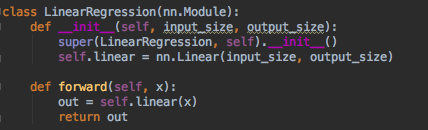# Manually initialize parameters?

Hi, I am newbie in pytorch.
Is there any way to initialize model parameters to all zero at first?
Say, if I have 2 input and 1 output linear regression, I will have 2 weight and 1 bias.
I want to make all weights and bias zero at first.
I couldn’t find other posts that deal with this issue.

1 Like

All at once…

``````for p in model.parameters():
p.data.fill_(0)
``````

Per layer

``````model.layer1.weight.data.fill_(0)
model.layer1.bias.data.fill_(0)``````
1 Like

It works well, thank you!

But this one doesn’t.
It says my model has no layer1 attribute.

I was assuming your model class declared a layer1 submodule.

Oh I didn’t.
Actually It is very as simple as below.Aha. You called it `.linear` rather than `.layer1`, so this will work…

``````model.linear.weight.data.fill_(0)
model.linear.bias.data.fill_(0)``````
1 Like

It is great!
Got some intuition about pytorch too.
Thank you!

1 Like

does the answer for this question need to be updated because I think I read somewhere that .data was going to be removed…is that correct?

For example the following code gives an error:

``````param.normal_(mean=mu,std=s)
RuntimeError: a leaf Variable that requires grad has been used in an in-place operation.
``````

I know that doing .data works but if I recall thats going to be removed

@albanD

Sorry to bug you, but what is the right way to `_fill` parameters given that the `.data` field might be going away (or is bad practice) or something like that I heard/read somewhere in the forum. What’s your take for a safe way to answer this question (i.e. for custom initialization of NNs)?